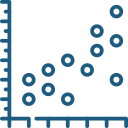Uh Oh! It seems you’re using an Ad blocker!

We always struggled to serve you with the best online calculations, thus, there's a humble request to either disable the AD blocker or go with premium plans to use the AD-Free version for calculators.

Or# Residual Calculator

Enter the independent and dependent variable in the tool and the calculator will find the residual value.

Independent variable X data, separated with comma (,):

Dependent variable Y data, separated with comma (,):

Table of Content

Get the Widget!

Add this calculator to your site and lets users to perform easy calculations.

Feedback

How easy was it to use our calculator? Did you face any problem, tell us!

The residual calculator calculates the residual of the independent variable (X) and dependent variable (Y) on the basis of linear regression.

The online residual point calculator can evaluate the error in the regression analysis.

## What is the Regression Residual?

The regression residual is the difference between the observed and predicted values in the regression model of the dataset.

The residual calculator provides accuracy and precision of the estimated results.

Actually, the residual find the margin of error of the dataset values by drawing the difference between the actual and forecasted values.

### How to Calculate the Residual?

The formula for the residual in statistics is given below:

###### Residual = Observed value – Predicted value

Where:

Observed value =  The actual values of the variable

Predicted value = The expected values of the variable

The residual graph calculators make sure what is the variance and the drift in the statistical data.

### Practical Example:

Now understand the concept of the residual by the practical example:

Let’s suppose there is a set of independent variables 1, 13, 5, 7, 9 and dependent variables 2, 4, 6, 18, and 10. Now the residuals for each observation in a simple linear regression model are given below:

#### Solution:

##### Dependent and Independent Variables:

The data set values for the dependent and independent variables are:

 Obs. X Y 1 1 2 2 13 4 3 5 6 4 7 18 5 9 10
##### The Regression Coefficient:

Now, construct the estimated regression coefficient using the values of the predicted and response variables:

 Obs. X Y Xᵢ² Yᵢ² Xᵢ · Yᵢ 1 1 2 1 4 2 2 13 4 169 16 52 3 5 6 25 36 30 4 7 18 49 324 126 5 9 10 81 100 90 Sum = 35 40 325 480 300
##### The Sum of the Squares Values:

The sum of the square generated from the above table are:

$$SS_{XX} = \sum^n_{i-1}X_i^2 – \dfrac{1}{n} \left(\sum^n_{i-1}X_i \right)^2$$

Now by step by step-by-step calculation:

= 80

$$SS_{YY} = \sum^n_{i-1}Y_i^2 – \dfrac{1}{n} \left(\sum^n_{i-1}Y_i \right)^2$$

= 160

$$SS_{XY} = \sum^n_{i-1}X_iY_i – \dfrac{1}{n} \left(\sum^n_{i-1}X_i \right) \left(\sum^n_{i-1}Y_i \right$$

= 20

##### The Slope of the Line:

$$hat{\beta}_1 = \dfrac{SS_{XY}}{SS_{XX}}$$

$$= \dfrac{20}{80}$$

$$= 0.25$$

$$hat{\beta}_0 = \bar{Y} – \hat{\beta}_1 \times \bar{X}$$

$$= 6.25$$

$$hat{Y} = 6.25 + 0.25X$$

##### The Predicted Values:

After finding the regression equation, we can gather the predicted values by inserting the independent variable in the regression equation.

$$hat{Y} = 6.25 + 0.25X$$

##### The Residual Values:

The predicted and residual values are given in the table below:

 Obs. X Y Predicted Values Residuals value=(Y-P.V) 1 1 2 6.25 + 0.25 × 1 = 6.5 2 – 6.5 = -4.5 2 13 4 6.25 + 0.25 × 13 = 9.5 4 – 9.5 = -5.5 3 5 6 6.25 + 0.25 × 5 = 7.5 6 – 7.5 = -1.5 4 7 18 6.25 + 0.25 × 7 = 8 18 – 8 = 10 5 9 10 6.25 + 0.25 × 9 = 8.5 10 – 8.5 = 1.5

## Working of Residual Calculator:

The online statistics residual calculator requires the values of the “X” and “Y” variables:

Let’s find out how!

Input:

• Enter the dependent and independent variables
• Tap Calculate

Output:

• The regression residual
• Step by Step calculations

## FAQs:

### Why Use the Residual Value?

The residual values are a good way to know the quality of the sample data. The main reason is that you are comparing the actual values with the expected values of certain phenomena. The online statistics residuals calculator increases the quality of the regression analysis.

## References:

From the source of NZmaths.co.nz: Residual, Linear regression

From the source of Originlab.com: Residual Plot, Checking error